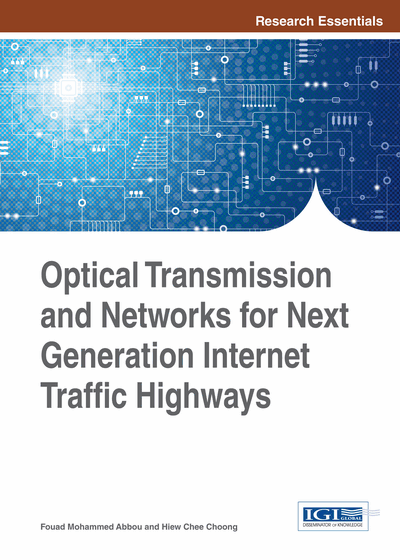# Phase Encoded Optical Code Division Multiplexing Access System

DOI: 10.4018/978-1-4666-6575-0.ch006

## Abstract

Phase encoded optical code division multiple-access system is evaluated in a dispersive fiber medium in this chapter. An approximate analytical expression for the root mean square (rms) width of the phase encoded signal (pseudorandom optical signal with low intensity) propagating in linear dispersive fibers is derived. Bit-Error Rate (BER) analysis of the system is performed in the case of both ordinary Single-Mode Fiber (SMF) and Dispersion-Shifted Fiber (DSF). The numerical results demonstrate that even though system performance improves due to the smaller width of initial Gaussian optical pulse, the effect from dispersion is higher. Larger code length reduces the effect of dispersion and the use of DSF greatly increases the transmission distance.
Chapter Preview
Top

## System Block Diagram And Description

A phase encoded OCDMA communication system can be modeled using the block diagram shown in Figure 1 (Chua, Abbou, Chuah, & Majumder, 2004). The transmitter consists of a pulse generator, a data generator and a spectral phase encoder. The pulse generator outputs an ultrashort pulse which is multiplied by the data generator. A data source takes on either “0” or “1” for on-off keying (OOK). When a data is “0”, the ultrashort pulse is not transmitted. While, when a data is “1”, the encoder multiplies the Fourier transform of the ultrashort pulse with the spectral phase code. An inverse Fourier transform then converts the resultant spectral waveform back to the temporal domain as a phase-encoded signal. The phase-encoded signal is transmitted over a fiber link, which we can assume is loss-compensated by a series of optical amplifiers. Fiber dispersion effects and interfering signal from other users (the MAI) are added onto the phase-encoded signal. The receiver consists of a conjugate spectral phase code generator, a photodetector, and data decision circuit. At the receiver, the phase code of the mth decoder is the conjugate code of the mth encoder.

Assume the pulse generator generates an initial Gauss optical pulse

(6.1)

The spectral shape of the pulse can be expressed as

(6.2)

Assume that the appendix phase to the spectrum by the spectral phase encoder can be written as (Ma, Zuo, Pu, & Lin, 2002):

(6.3)

## Complete Chapter List

Search this Book:
Reset